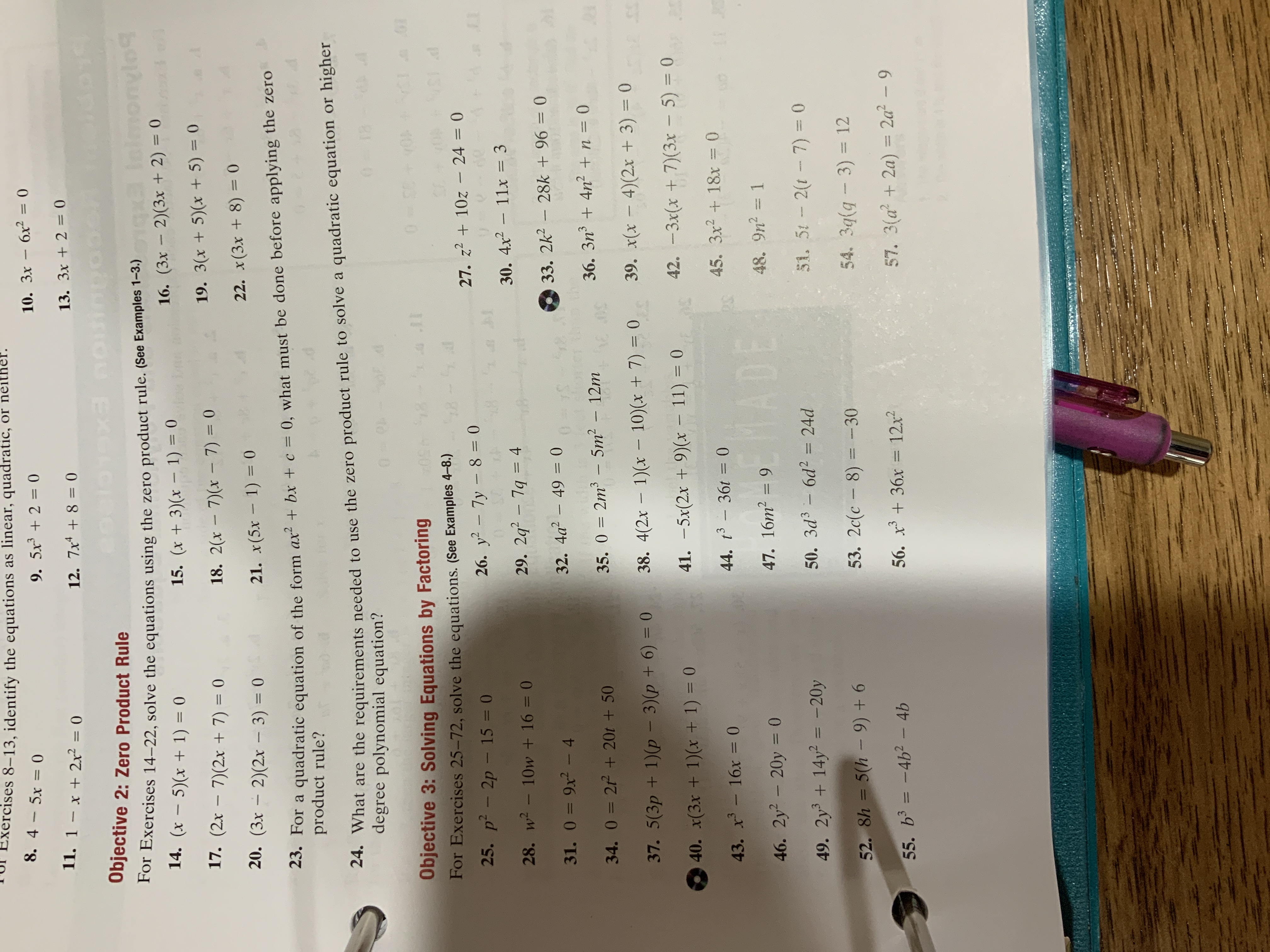# xercises 8-13, identify the equations as linear, quadratic, or neither. 8. 4 5x 0 9. 5x 2 0 10. 3x - 6x2 = 0 11. 1 - x + 2x = 0 12. 7x4 8= 0 13. 3x+2= 0 Objective 2: Zero Product Rule (s&opa For Exercises 14-22, solve the equations using the zero product rule. (See Examples 1-3.) 14. (x - 5) (x + 1) 16. (3x 2)(3x + 2) 0 bopuou 15. (x 3)x 1) = 0 1 0 17. (2x - 7)(2 + 7) = 0 18. 2x 7)(x 7) 0 19. 3(x+ 5)(x + 5) 0 20. (3x 2)(2x - 3) 0 21. x(5x - 1)0 0 (8 + xe)x "z 1 23. For a quadratic equation of the form ax bx + c = 0, what must be done before applying the zero product rule? 24. What are the requirements needed to use the zero product rule to solve a quadratic equation or higher degree polynomial equation? o 0 Objective 3: Solving Equations by Factoring E0 17 D 1 For Exercises 25-72, solve the equations. (See Examples 4-8.) P 13h 27. z210z - 24 = 0 25. p2- 2p - 15 0 26. y2 - 7y - 8= 0 TM S 30. 4x2 11x = 3 17 28. w2- 10w + 16 = 0 29. 2q 7q 4 33. 2k2 28k +96 = 0 31. 0=9x2-4 32. 4a 49 0 34. 0=2P+20r+ 50 35. O =2m3 - 5m2 - 12m 36. 3n 4n n = 0 S0 39. x(x - 4)(2x +3) = 0 37. 5(3p+1)(p- 3)(p + 6) 0 38. 4(2x 1)(x - 10)(x + 7) = 0 3T 30 40. x(3x + 1)(x + 1) = 0 41. -5x(2x + 9)(x 11) 0 42. -3x(x + 7)(3x 5) = 0 43. x 16x = 0 44. t3- 36t= 0 45. 3x2 18x = 0 O 46. 2y2 20y 0 47. 16m2 =9 48. 9n2 1 1 30YM 49. 2y+ 14y2 -20y 50. 3d3 6d2 = 24d 51. 5t 2(t-7) = 0 52. 8h 5(h-9)+6 53. 2c(c 8) - 30 54. 3q(g 3) 12 = 55. b3 = -4b2 -4b 56. x36x12x2 57. 3(a2a)= 2a- 9

Question

I did this one #27help_outlineImage Transcriptionclosexercises 8-13, identify the equations as linear, quadratic, or neither. 8. 4 5x 0 9. 5x 2 0 10. 3x - 6x2 = 0 11. 1 - x + 2x = 0 12. 7x4 8= 0 13. 3x+2= 0 Objective 2: Zero Product Rule (s&opa For Exercises 14-22, solve the equations using the zero product rule. (See Examples 1-3.) 14. (x - 5) (x + 1) 16. (3x 2)(3x + 2) 0 bopuou 15. (x 3)x 1) = 0 1 0 17. (2x - 7)(2 + 7) = 0 18. 2x 7)(x 7) 0 19. 3(x+ 5)(x + 5) 0 20. (3x 2)(2x - 3) 0 21. x(5x - 1)0 0 (8 + xe)x "z 1 23. For a quadratic equation of the form ax bx + c = 0, what must be done before applying the zero product rule? 24. What are the requirements needed to use the zero product rule to solve a quadratic equation or higher degree polynomial equation? o 0 Objective 3: Solving Equations by Factoring E0 17 D 1 For Exercises 25-72, solve the equations. (See Examples 4-8.) P 13h 27. z210z - 24 = 0 25. p2- 2p - 15 0 26. y2 - 7y - 8= 0 TM S 30. 4x2 11x = 3 17 28. w2- 10w + 16 = 0 29. 2q 7q 4 33. 2k2 28k +96 = 0 31. 0=9x2-4 32. 4a 49 0 34. 0=2P+20r+ 50 35. O =2m3 - 5m2 - 12m 36. 3n 4n n = 0 S0 39. x(x - 4)(2x +3) = 0 37. 5(3p+1)(p- 3)(p + 6) 0 38. 4(2x 1)(x - 10)(x + 7) = 0 3T 30 40. x(3x + 1)(x + 1) = 0 41. -5x(2x + 9)(x 11) 0 42. -3x(x + 7)(3x 5) = 0 43. x 16x = 0 44. t3- 36t= 0 45. 3x2 18x = 0 O 46. 2y2 20y 0 47. 16m2 =9 48. 9n2 1 1 30YM 49. 2y+ 14y2 -20y 50. 3d3 6d2 = 24d 51. 5t 2(t-7) = 0 52. 8h 5(h-9)+6 53. 2c(c 8) - 30 54. 3q(g 3) 12 = 55. b3 = -4b2 -4b 56. x36x12x2 57. 3(a2a)= 2a- 9 fullscreen

### Want to see this answer and more?

Experts are waiting 24/7 to provide step-by-step solutions in as fast as 30 minutes!*